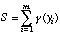# Manual Page for Command >>> QUADRATIC-LINEAR

Syntax

Parent Command
>> OPTION

Subcommand
-

Description
This command selects a quadratic-linear objective function. Given this estimator, the objective function to be minimized is a combination of least-squares for small residuals and the first norm for residuals larger than c-times the prior standard deviation:wherewithThis objective function does not correspond to a standard probability density function. It has the general characteristic that the weight given individual residuals first increases quadratically with deviation, then only linearly to reduce the impact of outliers. For c –> infinity, the estimator is identical to least-squares; for c –> 0, it approaches the L1-estimator. Note that this objective function is minimized using the standard Levenberg-Marquardt algorithm which is designed for a quadratic objective function. Since the function is quadratic for y < c, the Levenberg-Marquardt algorithm is usually quite efficient.

Example
> COMPUTATION
>> OPTION
>>> use a QUADRATIC-LINEAR robust estimator with a constant c : 1.0
<<<
<<